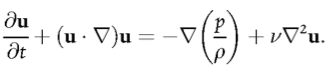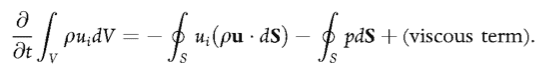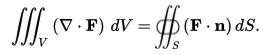# Integral form of Navier-Stokes Equation

• I
The Navier-Stokes equation may be written as:If we have a fixed volume (a so-called control volume) then the integral of throughout V yields, with the help of Gauss' theorem:(from 'Turbulence' by Davidson).

The definition of Gauss' theorem:Could someone show me how to go from the integral over the control volume to the closed surface integrals on the left of the second equation? Do we need to use Leibniz's integral rule or Reynolds Transport theorem?

Chestermiller
Mentor
The Navier-Stokes equation may be written as:
View attachment 101838

If we have a fixed volume (a so-called control volume) then the integral of throughout V yields, with the help of Gauss' theorem:
View attachment 101837
(from 'Turbulence' by Davidson).

The definition of Gauss' theorem:
View attachment 101836

Could someone show me how to go from the integral over the control volume to the closed surface integrals on the left of the second equation? Do we need to use Leibniz's integral rule or Reynolds Transport theorem?
I think you meant "right", not "left". The first equation assumes that the density is constant, so you can multiply both sides by ##\rho##. You have the identity:
$$\rho\vec{u}\cdot \nabla \vec{u}+\rho \vec{u}\nabla \cdot \vec{u}=\nabla \cdot (\rho \vec{u} \vec{u})$$
But, for an incompressible fluid, the second term on the left is equal to zero. Therefore, $$\rho\vec{u}\cdot \nabla \vec{u}=\nabla \cdot (\rho \vec{u} \vec{u})$$
Does this help?

I think you meant "right", not "left". The first equation assumes that the density is constant, so you can multiply both sides by ##\rho##. You have the identity:
$$\rho\vec{u}\cdot \nabla \vec{u}+\rho \vec{u}\nabla \cdot \vec{u}=\nabla \cdot (\rho \vec{u} \vec{u})$$
But, for an incompressible fluid, the second term on the left is equal to zero. Therefore, $$\rho\vec{u}\cdot \nabla \vec{u}=\nabla \cdot (\rho \vec{u} \vec{u})$$
Does this help?

I have not encountered this identity. Secondly, the RHS of the identity has two vectors multiplied, which I don't understand since vector multiplication is either dot product, cross product or combinations thereof?

Last edited:
Chestermiller
Mentor
Firstly, I can't see why the author chose to represent the material derivative as (U.∇)U - I've previously always encountered it as U.(∇U), which makes more intuitive sense to me. Clearly these must be the same, but I can't see how?
Write it out in component form for Cartesian coordinates and you will see how.
Secondly, I have not encountered this identity.
Again, write it out in component form for Cartesian coordinates.
Thirdly, the RHS of the identity has two vectors multiplied, which I don't understand since vector multiplication is either dot product, cross product or combinations thereof?
I guess you are not familiar with dyadic tensor notation. A dyad consists of two vectors placed in juxtaposition, without any operation such as dot product or cross product implied between the two. Such an entity is a 2nd order tensor. In this case, the tensor ##\rho \vec{u} \vec{u}## represents the momentum flux per unit volume of fluid. To learn more about the powerful use of dyadic tensor notation and the implementation of mathematical operations using dyadics, see Transport Phenomena by Bird, Stewart, and Lightfoot (Appendices). In my judgment, it is something very worthwhile to learn by those learning and using Fluid Mechanics.

By the way, when you wrote ##(\vec{\nabla} \vec{u})##, you were already employing a dyadic of the vector operator ##\vec{\nabla}## and the vector ##\vec{u}##.

•FluidStu
vanhees71
Gold Member
2021 Award
Chestermiller is obviously using a tensor notation with the abbreviation ##\vec{u} \vec{u}=\vec{u} \otimes \vec{u}##. It's easier in components. The term in the NS equation we are discussing we have (in Cartesian coordinates)
$$(\vec{u} \cdot \vec{\nabla} \vec{u})_j=u_k \partial_k u_j=\partial_k(u_k u_j)-u_j \partial_k u_k.$$
Now obviously, it's tacidly assumed that the fluid is incompressible and thus
$$(\vec{u} \cdot \vec{\nabla} \vec{u})_j = \partial_k(u_k u_j),$$
i.e., a total divergence that can be transformed to a surface term when integrating over ##V##.

What's also used is the continuity equation
$$\partial_t \rho+\vec{\nabla}(\rho \vec{v})=0.$$

•FluidStu